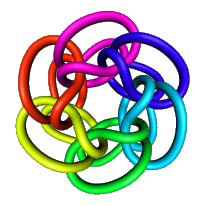BackA survey of some of the important applications of calculus and matrix algebra Topics include model fitting, experimental modeling, modeling with systems of differential equations, and optimization (3 credits) Prerequisite: Min. "C" in both MATH 200 and MATH 241

Course Objective:

This course is designed to provide the students with a fundamental understanding of how Mathematics is applied as a tool to aid in studying complex systems in science and engineering. It will help a student to overcome the difficult transition from basic assumptions made on a real problem to the setting up of a model in mathematical terms. There would be no place in this course where a student could ask, "What is all of this for?" We will introduce modern technology such as Maple and Matlab. When you have gone through the course, you will have learned how to tackle real-world problems.

This course presents a wide range of well-developed and actual models from Ecology, Biology, Medicine, Business and Economics, and  Engineering. This selection will illustrate how Mathematics can help us to understand the patterns of life in Nature.

Comments on the solutions and revisions to the models will be important aspects.

Syllabus:

The course is designed around some topics such as:

· Fitting models to data graphically and analytically. Choosing the best model.

· Harvesting strategies. Smoothing with polynomial and spline models

· Dynamics in interacting multiple species populations. Prey-predator systems (e.g. Lotka-Volterra models) and their applications in Ecology.

· Models for the spread of infectious diseases. Application to modelling the spread of the HIV virus. Outbreaks(e.g. SARS, West Nile Virus, etc)

· Discrete and Continuous optimization modeling

· Managing Renewable Resources. The Fishing Industry.

Skills  and Knowledge Acquired:

Understanding of single-variable calculus and linear algebra.  Motivation and appreciation some of the difficulties and successes of mathematical modelling and use of the modern technology.

40% Final Exam
24% Six Assignments:

1.Difference Equations         Chapter 1

2. Differential Equations      Chapter 10

3. System of ODE              Chapter 11

4. Model Fitting                   Chapter 3

5. Discrete Optimization      Chapter 7

6. Continuous Optimization Chapter 12

11% Group Project
25% Individual Project

Text: A First Course in Mathematical Modeling, Third Edition, by F.R. Giordano, M.D. Weir, and W.P. Fox

Important Dates:

· TBA –Group Project Report

· TBA –Individual Project Report

·  FINAL EXAM  TBA

Sample test

Projects

A suggested format for the project report is:

1. brief introduction

2. description of the formulation of the model

3. discussion of numerical methods

4. results and investigation of model (Results should be presented as graphs or tables)

Group Project

This project should be on one of the following topics:

1. Difference Equations      Chapter 1

2. Differential Equations      Chapter 10

3. System of ODE                 Chapter 11

Individual Project

You may do the project on any topic with the following restriction: you should use a modelling approach that is different from the one you used for the group project.

Library Cat.:

QH 541.15 M3 C467 2003
Cushing, J. M. Chaos in ecology : experimental nonlinear dynamics

QH 352 T45 2003
Thieme, Horst R., Mathematics in population biology

PAM 2049
Hilke, Eileen Veronica, Mathematical modelling

QR 182.2 M36 M372
Marchuk, G. I. Mathematical modelling of immune response in infectious diseases

QA 401 M5138
Mesterton-Gibbons, Michael , A concrete approach to mathematical modelling

QA 401 M48

Mark Meerschaert,  "Mathematical Modeling",

QA 401 G88

D. Edwards and M. Hamson,  "Guide to Mathematical Modeling",

QA 401 S214

J.G. Andrews and R.R. McLone , "Mathematical modelling",

QA 401 S214

T.L. Saaty and J.M. Alexander , "Thinking with models",

QA 371 B795
Braun, Martin, "Differential equations and their applications : an introduction to applied mathematics"

QH 352 B73
Brauer, Fred "Mathematical models in population biology and epidemiology"

QA 401 G88
Edwards, Dilwyn,"Guide to mathematical modelling"

PostCALC Project

Interesting Facts about Population Growth Mathematical Models

Jianhong Wu's homepage!

Gerda de Vries @ Work

Avoiding Mistakes in Population Modeling

http://nlds.sdsu.edu/index.html#vortex

http://www.arcytech.org/java/population/facts_math.html

http://www.gypsymoth.ento.vt.edu/~sharov/alexei.html

http://www.mec.utt.ro/~petrisor/dynsyst.html

http://www.vrvis.at/vis/resources/diss-LM/node75.html

http://www.sci.wsu.edu/idea/Logistic/ecology.html